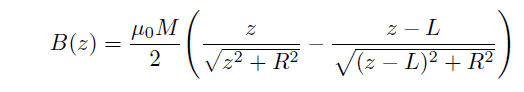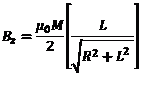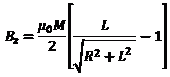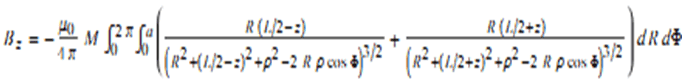# Cylindrical magnet

umby
Hi, I am looking for the formula of the magneti field along the axis of a axially magnetized cylindrical magnet.
Unfortunately, there are quite different answers on Internet.
Is the uploaded formula (where R is the magnet radius and L its length) correct?#### Attachments

Homework Helper
Gold Member
2021 Award
It looks right. If I were you, I would go along the more complete equation (8) given here
http://web.mit.edu/6.013_book/www/chapter9/9.3.html
because it provides the field inside the magnet as well. Note that this equation has the mid point of the magnet as the origin, unlike yours where the origin is at one of the faces.

umby
Thank you very much. However, eq. (8) does not have an anlytical solution, does it? You need to use numerical integration to solve it.

Homework Helper
Gold Member
2021 Award
It is an analytical solution. Perhaps you confused the ##d~M_0## up front for a differential. It is not; ##d## is the length of the cylinder as shown in the drawing and ##M_0## is the magnitude of the magnetization.

umby
Ohh, I did!
Thank you so much.
Is a 1/2 the value subtracted to z/d?

Homework Helper
Gold Member
2021 Award
Actually I see that the two formulae are different. Indeed, replacing d with L and changing the origing z = z1-d/2 in eq.(8), you get:
View attachment 226284
Right.

Homework Helper
Gold Member
2021 Award
So μ0 is replaced by d in your formula.
No. If you follow the proof in the MIT link, you will see that μ0 drops out in equation (6). The symbol ##d## is the half-length of the cylinder. In other words ##2d=L##.
IS M0 measured in A/m?
Yes.

umby
I have a doubt: going back to eq. (8), the term in the square brackets is adimensional, M0 is [A/m] times d[m], you get a Hz in [A].

Homework Helper
Gold Member
2021 Award
I have a doubt: going back to eq. (8), the term in the square brackets is adimensional, M0 is [A/m] times d[m], you get a Hz in [A].
You are correct. I re-derived the whole thing and equation (8) should have ##\frac{M_0}{2}## as the overall factor without the ##d##. The ##d## in the numerator cancels out when one makes the denominator dimensionless. Whoever posted this probably missed the cancellation, but this goes to show that you should distrust what you see on the web, even if it's from MIT.

umby
Thank you very much. Not distrust, but re-think critically.
Finally I have the field thanks to your help.
Last question: I can swop from B to H with μ0, but if I need the force on a particle in the field of the magnet, I have to use B or H?

Homework Helper
Gold Member
2021 Award
Thank you very much. Not distrust, but re-think critically.
Finally I have the field thanks to your help.
Last question: I can swop from B to H with μ0, but if I need the force on a particle in the field of the magnet, I have to use B or H?
I would say use B. What equation do you plan to use to find the force?

umby
The classical derivation from the analogy with the force on an electric dipole: (m*Del) B.

Kuruman, please, do you know a complete expression for the field of a cylindrical magnet, not only along the axis of the magnet?

Homework Helper
Gold Member
2021 Award
The classical derivation from the analogy with the force on an electric dipole: (m*Del) B.

Kuruman, please, do you know a complete expression for the field of a cylindrical magnet, not only along the axis of the magnet?
An analytical expression for that involves elliptic integrals so I don't think there is one. If the force is to be calculated very near the z-axis you may do a series expansion of the radial field component. If not, then a numerical calculation may be appropriate.

umby
And and expression to calculate numerically it? Do you know a link in the web?

Homework Helper
Gold Member
2021 Award
Sorry, I don't know of any links. I am sure you can research the web as well as me, if not better.

umby
Applying the two formulae, which we have discussed so far, it is easy to calculate the z-component of field at the top of the magnet along its axis:Now, I found a page of Mathematica by Wolfram, which reports an expression, to be computed numerically, for the whole field:
http://demonstrations.wolfram.com/MagneticFieldOfACylindricalBarMagnet
However, this expression does not converge to the above one for ρ -> 0 and z ->L/2.
Do you have the same result?

#### Attachments

Homework Helper
Gold Member
2021 Award
Applying the two formulae, which we have discussed so far, it is easy to calculate the z-component of field at the top of the magnet along its axis:
View attachment 226332
Now, I found a page of Mathematica by Wolfram, which reports an expression, to be computed numerically, for the whole field:
http://demonstrations.wolfram.com/MagneticFieldOfACylindricalBarMagnet
However, this expression does not converge to the above one for ρ -> 0 and z ->L/2.
Do you have the same result?
To me the Wolfram expression looks like the same as the integral leading to the corrected MIT expression. I don't understand what you mean by ρ→0. ρ is an integration variable. If you want to see what happens when you have a long thin cylindrical magnet, first you integrate then you look what happens in the limit R/L <<1.

umby
The integration variables are R and Φ. R integrated over [0, a] and Φ over [0, 2 Pi].
After the integration, you have the field in generic ρ and z, namely Bz(ρ,z).
I want the field in (ρ = 0, z =L/2), i.e. Bz(0,L/2).

Homework Helper
Gold Member
2021 Award
The integration variables are R and Φ. R integrated over [0, a] and Φ over [0, 2 Pi].
After the integration, you have the field in generic ρ and z, namely Bz(ρ,z).
I want the field in (ρ = 0, z =L/2), i.e. Bz(0,L/2).
Yes you are correct. I was thinking of the other two expressions where R is the radius of the cylinder. I have not done it, but it looks like if you set φ=0 and do the φ integral (i.e. stick in a 2π) in the Wolfram expression, you should get equation (6) in the MIT link. Have you tried that?

umby
This is the problem, you do not get the MIT expression for Bz(0,L/2, "or the surface of the magnet") but:While the MIT expression is:#### Attachments

Homework Helper
Gold Member
2021 Award
Hz is discontinuous at the surface. Look at the plot in the MIT link below equation (5).

umby
Hz is discontinuous at the surface. Look at the plot in the MIT link below equation (5).
I mean the field on the magent Surface coming from the outside of the magnet

Homework Helper
Gold Member
2021 Award
There is an expression for Hz below the surface (u = 2 in equation 8) and another expression above the surface (u = 0 in equation 8). The expression is double-valued on the surface because Hz is discontinuous across the surface. If you want to compare expressions, you must be on the same side of the surface.

umby
There is an expression for Hz below the surface (u = 2 in equation 8) and another expression above the surface (u = 0 in equation 8). The expression is double-valued on the surface because Hz is discontinuous across the surface. If you want to compare expressions, you must be on the same side of the surface.

Yes, on the same side of the Surface, u=0. In this way I did the calculation: I took the expression provided for Bz in Wolframand I set ρ = 0, z =L/2. I did the integration, to get the result:Then I took the MIT corrected (with u = 0) or my expression and I get:#### Attachments

Homework Helper
Gold Member
2021 Award
By setting z = L/2 before you do the integral, you lose the "inside - outside" information. Do the integral first, then set z = L/2 and you will see what I mean. At the lower limit the first integral will have a term ##\sqrt{(z-L/2)^2}##. This is ##z-L/2## when ##z>L/2## and ##L/2-z## when ##z<L/2##. If you prematurely set ##z=L/2##, you end up with zero in either case and you lose the discontinuity.

•sysprog
umby
By setting z = L/2 before you do the integral, you lose the "inside - outside" information. Do the integral first, then set z = L/2 and you will see what I mean. At the lower limit the first integral will have a term ##\sqrt{(z-L/2)^2}##. This is ##z-L/2## when ##z>L/2## and ##L/2-z## when ##z<L/2##. If you prematurely set ##z=L/2##, you end up with zero in either case and you lose the discontinuity.
Right!!

•sysprog
sysprog
^^^^^ a very nice, cogent, entertaining discussion ^^^^^ thanks

umby
By setting z = L/2 before you do the integral, you lose the "inside - outside" information. Do the integral first, then set z = L/2 and you will see what I mean. At the lower limit the first integral will have a term ##\sqrt{(z-L/2)^2}##. This is ##z-L/2## when ##z>L/2## and ##L/2-z## when ##z<L/2##. If you prematurely set ##z=L/2##, you end up with zero in either case and you lose the discontinuity.

I have just checked, it gives exactly the corrected MIT expression!!!